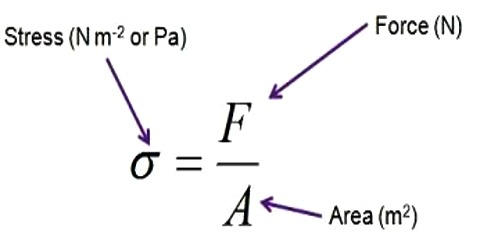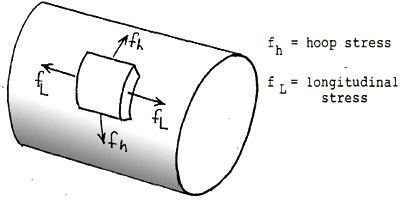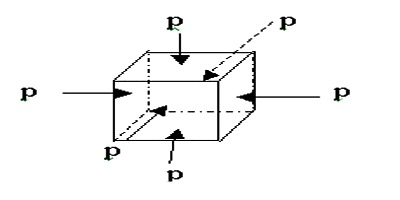Physics

# Describe Types of StressesTypes of stresses

Longitudinal stress: If the stress is normal to the surface, it is called normal stress. Stress is always normal in the case of a change in length or a wire or in the case of change in volume of a body. Restoring force per unit area perpendicular to the surface is called longitudinal or normal stress. Let the area of cross section of a wire be A. If a force F is applied along the length of the wire, then longitudinal stress = Force / Area = F/AShearing stress: The stress applied to produce shearing strain is called shearing stress. When the Stress is tangential to the surface due to the application of forces parallel to the surface, then the stress is called tangential or shearing stress. It changes the shape of the body. If force F is applied tangentially on the area A of an object and if there is a shearing strain due to this force, then shearing stress = Force / Area = F/AVolume stress: The stress applied to produce volume strain of a body is called volume stress. When a normal stress changes the volume of a body then it is called volume stress. When a solid body is immersed in a fluid, the force at any point is normal to the surface of the body and the magnitude of the force on any small area is proportional to the area i.e., the body is under the action of a pressure P. Let the volume strain be produced on an object by applying force F normal to all surfaces of the object. If A be the area of the surface, the volume stress = Force / Area = F/A.